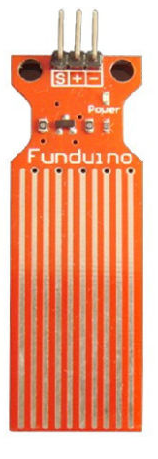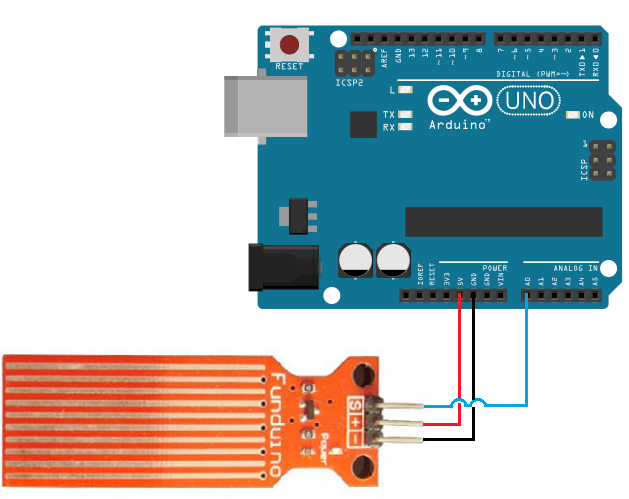﻿ How to Build a Liquid Level Sensor Circuit with an Arduino ﻿# How to Build a Liquid Level Sensor Circuit with an ArduinoIn this project, we will build a liquid level sensor circuit with an arduino.

This circuit will be able to tell us roughly how much of the sensor is covered by liquid.

Liquid level sensors are used for all types of applications. They are extensively used within automobiles, which rely on a substantial amount of different fluids in order to operate to check for how much gas in the car, windshield washer fluid, oil levles. Basically, they are used whenever we want to measure the level of any type of fluid of a system. Thus, they are extremely valuable to be able to learn and manipulate and build circuits with.

The liquid level sensor we will use is an analog sensor, meaning it outputs an analog voltage in proportion to the amount of liquid the sensor is exposed to. We just connect the analog pin, represented by an S, to an analog pin on the arduino board to read the analog value.

As you can see from the above image, the sensor has a series of parallel wires across the board. These wires are what sense the liquid level that the board is exposed to.

With our liquid level sensor and an arduino, there are a number of options of what we can do. We can use the sensor, simply, to read and output the analog value obtained. Or we can create a type of alarm indicator status system. For example, we can have a green LED light up when the sensor is completely full (submerged to the top with fluid), indicating that it's full. We can have a red LED light up when the sensor's liquid level falls below 1/4 level, like how car dashboard LED indicators tell us when our gas tank goes below E (empty).

So with a microcontroller like the arduino, there are basically limitless options of how we can incorporate the liquid level sensor.

In this circuit, we will build now, we will just do the most basic circuit and simply read and output the analog value read by the arduino.

Components Needed

• Arduino
• Liquid Level Sensor

The liquid level sensor we will use is built by China Harbin Okumatsu Robot Technology Co and its product item is RB-02S048. This part can easily be obtained on ebay for most of the time under \$2 including shipping. It's very inexpensive.

The sensor operates on 5V and needs less than 20mA for operating power current, which means the arduino can easily provide this (so no external power is needed to power it).

The liquid level sensor has 3 pins. It's very basic. The pinout is shown below.2 of the pins are for power, 1 connecting to the +5V of the arduino and the other connecting to the ground terminal of the arduino. The other pin, with an S, is the signal pin. This is the pin that outputs the analog voltage signal in proportion to the amount of the sensor which is covered with liquid. This pin connects to an analog pin on the arduino board to be read.

### Arduino Liquid Level Sensor Circuit

The schematic diagram of the arduino liquid level sensor circuit is shown below.This circuit is as basic as it can get.

The liquid level sensor board needs about 5V of power.

The signal pin (S) gets connected to an analog pin on the arduino board. Here we connect it to analog pin A0. This allows the arduino board to be able to read the analog voltage value.

### Code

With the hardware setup, all we need now is the code to obtain the sensor reading.

/*Code for Liquid Level Sensor Circuit Built with an Arduino*/

const int sensorPin= 0; //sensor pin connected to analog pin A0
int liquid_level;

void setup() {
Serial.begin(9600); //sets the baud rate for data transfer in bits/second
pinMode(sensorPin, INPUT);//the liquid level sensor will be an input to the arduino
}

void loop() {
Serial.println(liquid_level);//prints out liquid level sensor reading
delay(100);//delays 100ms
}

In the first block of code, we declare the sensorPin variable and initialize it to 0. We then declare a liquid_level variable, which will hold the value of the analog value output from the sensor, serving as our sensor reading, which represents the liquid level.

In our setup() function, we set the baud rate and make the sensorPin input, since it is an input value into the arduino board to be read.

In our loop() function, which is the code that repeats over and over, we read the value from the sensorPin and store it in the variable liquid_level. We then output this value to the Serial Monitor to be read. The code is constantly running this code over and over, so it obtains new readings endlessly. We give a delay of 100ms in between each reading to create a small pause in between.

Related Resources